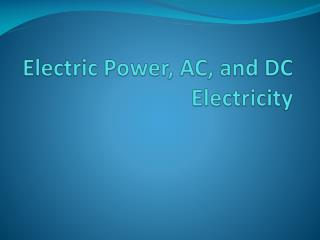Download PresentationElectric Power, AC, and DC Electricity

Loading in 2 Seconds...

# Electric Power, AC, and DC Electricity - PowerPoint PPT Presentation

Electric Power, AC, and DC Electricity. Key Question: How much does electricity cost and what do you pay for?. Electric Power, AC, and DC Electricity. *Students read Section 20.3 AFTER Investigation 20.3. Electric Power, AC, and DC Electricity. The watt (W) is a unit of power.I am the owner, or an agent authorized to act on behalf of the owner, of the copyrighted work described.
Download Presentation## Electric Power, AC, and DC Electricity

An Image/Link below is provided (as is) to download presentation

Download Policy: Content on the Website is provided to you AS IS for your information and personal use and may not be sold / licensed / shared on other websites without getting consent from its author.While downloading, if for some reason you are not able to download a presentation, the publisher may have deleted the file from their server.

- - - - - - - - - - - - - - - - - - - - - - - - - - E N D - - - - - - - - - - - - - - - - - - - - - - - - - -
Presentation Transcript
1. Electric Power, AC, and DC Electricity

2. Key Question: How much does electricity cost and what do you pay for? Electric Power, AC, and DC Electricity *Students read Section 20.3 AFTER Investigation 20.3

3. Electric Power, AC, and DC Electricity • The watt (W) is a unit of power. • Power is the rate at which energy moves or is used. • Since energy is measured in joules, power is measured in joules per second. • One joule per second is equal to one watt.

4. Reviewing terms

5. Power in electric circuits • One watt is a pretty small amount of power. • In everyday use, larger units are more convenient to use. • A kilowatt (kW) is equal to 1,000 watts. • The other common unit of power often seen on electric motors is the horsepower. • One horsepower is 746 watts.

6. Power Voltage (volts) P = VI Current (amps) Power (watts)

7. Calculate power • A light bulb with a resistance of 1.5Ω is connected to a 1.5-volt battery in the circuit shown at right. • Calculate the power used by the light bulb.

8. Paying for electricity • Electric companies charge for the number of kilowatt-hours used during a set period of time, often a month. • One kilowatt-hour (kWh) means that a kilowatt of power has been used for one hour. • Since power multiplied by time is energy, a kilowatt-hour is a unit of energy. • One kilowatt-hour is 3.6 x 106 joules.

9. Calculate power • Your electric company charges 14 cents per kilowatt-hour. Your coffee maker has a power rating of 1,050 watts. • How much does it cost to use the coffee maker one hour per day for a month?

10. Alternating and direct current • The current from a battery is always in the same direction. • One end of the battery is positive and the other end is negative. • The direction of current flows from positive to negative. • This is called direct current, or DC.

11. Alternating and direct current • If voltage alternates, so does current. • When the voltage is positive, the current in the circuit is clockwise. • When the voltage is negative the current is the opposite direction. • This type of current is called alternating current, or AC.

12. Alternating and direct current • AC current is used for almost all high-power applications because it is easier to generate and to transmit over long distances. • The 120 volt AC (VAC) electricity used in homes and businesses alternates between peak values of +170 V and -170 V at a frequency of 60 Hz. • AC electricity is usually identified by the average voltage, (120 VAC) not the peak voltage.

13. Application: Wiring in Homes and Buildings

14. Application: Wiring in Homes and Buildings